# DU = TdS - p dV is valid for irreversible process -- Proof

• I
Pushoam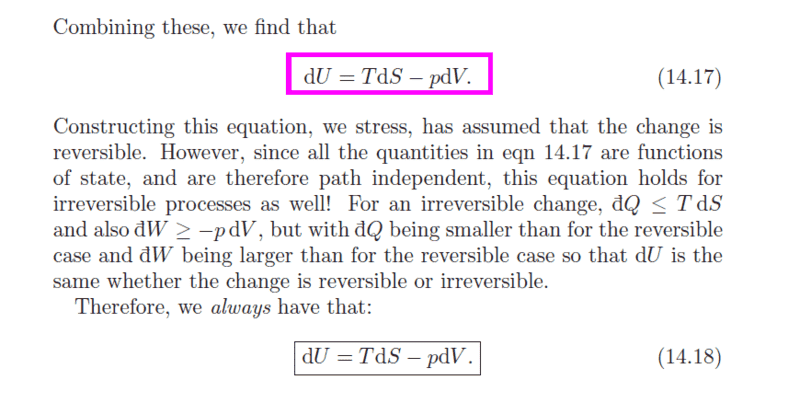The book says that all quantities in eq. 14.17 are state- functions. How do we know that?
Earlier I read that both W and Q are not state functions.
Now, since S is state-function, it means that tem. is not a state- function, isn't it?
Does it mean that for a reversible process, both W and Q are state-functions? How to prove it?
How can we have eq. 14.18 always as entropy is not defined for irreversible process?

Staff Emeritus
Homework Helper
Gold Member
A state function is a function of the state the system is in. Work and heat are not state functions because they depend on how the system is changed (the path the system takes in the state space).

Pushoam
A state function is a function of the state the system is in. Work and heat are not state functions because they depend on how the system is changed (the path the system takes in the state space).
dS is a state function for reversible process. It is not defined for irreversible process. So, how can the eqn 14.18 hold true for irreversible process, too?
This is what I want to know.

Staff Emeritus
Homework Helper
Gold Member
dS is a state function for reversible process.
dS is not a state function. It is the differential of a state function.

Pushoam
dS is not a state function. It is the differential of a state function.
Yes, sorry for that. What I want to say was:
S is a state function for reversible process. It is not defined for irreversible process. So, how can the eqn 14.18 hold true for irreversible process, too?
This is what I want to know.

Staff Emeritus
Homework Helper
Gold Member
S is a state function for reversible process.
No, a state function does not depend on any process. It is just a function of the state of the system, not of how the system develops.

Pushoam
No, a state function does not depend on any process. It is just a function of the state of the system, not of how the system develops.
Yes, but S is defined only for a reversible process. As only for a reversible process, ##\oint\frac{dQ_{rev}}T = 0##. So, the concept of S is defined only for reversible process.

Staff Emeritus
Homework Helper
Gold Member
Yes, but S is defined only for a reversible process.
No, S is defined only for a state. It has nothing to do with any process of any sort.

Pushoam
No, S is defined only for a state. It has nothing to do with any process of any sort.
But for an irreversible process, ##\oint \frac{dQ} T <0 ## . So, S is not a state- function for an irreversible process.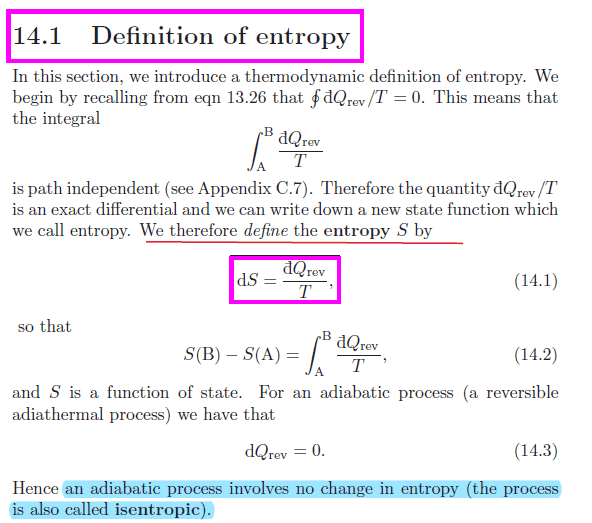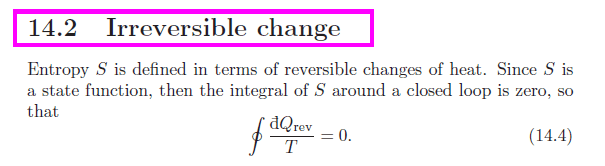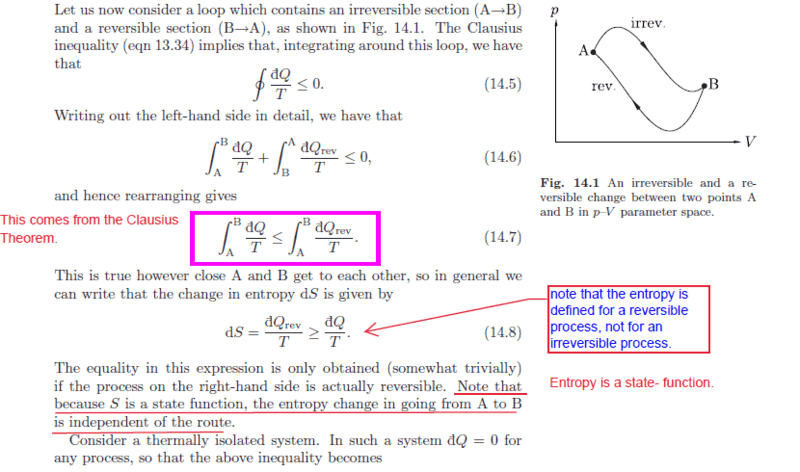What I have understood is:

The concept of entropy is defined :
dS = ##\frac {dQ_{rev}} T## such that ##\oint dS = 0## i.e. S is a state-function.

Even though the system is going through an irreversible process we can talk of entropy of the system as ## Q_rev## could be defined assumng that the system is in some reversible process. Of course, for this purpose, all reversible processes become equivalent as S depends only on the state of the system .

In the following eqn.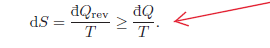if the system is in the reversible process, then ##dQ = dQ_{rev}##.
For example, if the system has dQ =0, i.e. thermally isolated and
a) it is in the reversible process, then dS = 0 ,
otherwise i.e.
B) the system is in the irreversible process, then ##dQ_{rev} > [dQ =0]~,dQ_{rev} >0##.

Now, my question is this ,
If the system is thermally isolated, then dQ =0 (from the definition of thermal isolation).
So, whether the system goes through reversible or irreversible process, dQ remains 0
i.e. dQ remains 0 independent of the process the system undergoes through. So, in this case, ##dQ_{rev} = dQ_{irrev}##. Right?
Is this correct?

#### Attachments

Pushoam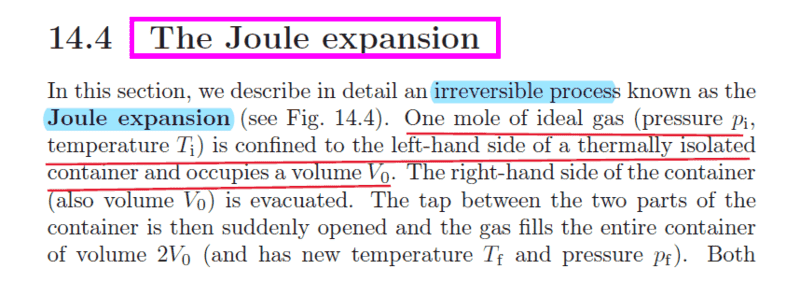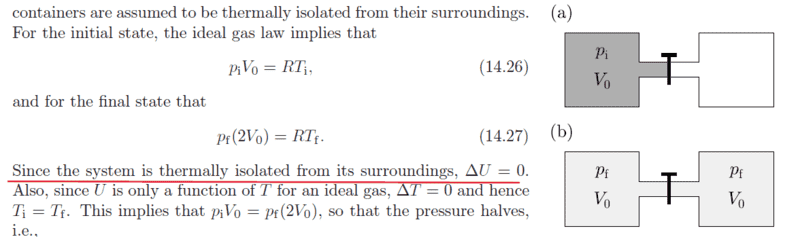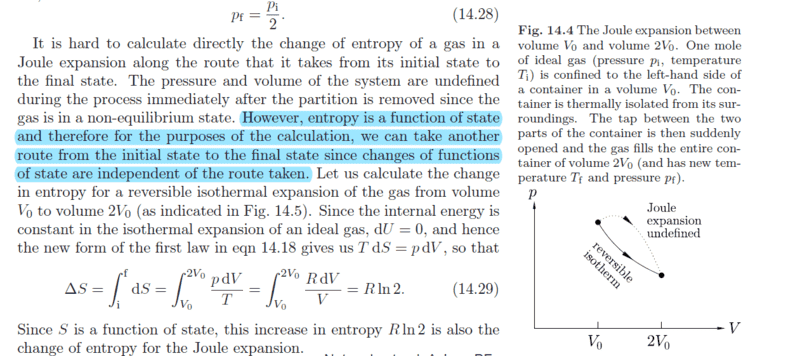Since, Joule expansion itself is an irreversible, the path followed by the system is undefined.
So, for calculating entropy change, we simply find out the previous and the final state i.e.(##p_i, V_i, T_i) ~ and ~ (p_f,V_f,T_f).##
We define a reversible process which leads the system from the initial state to the final state. Any reversible process would work as ##\Delta##S depends only on the initial and final state.
Here, isothermal expansion acts as the suitable reversible process as the tem. remains the same (in both final and initial state).
Then, using the reversible process and 1st Law of thermodynamics with entropy, we calculate ##\Delta S##.
Is my understanding correct?

Pushoam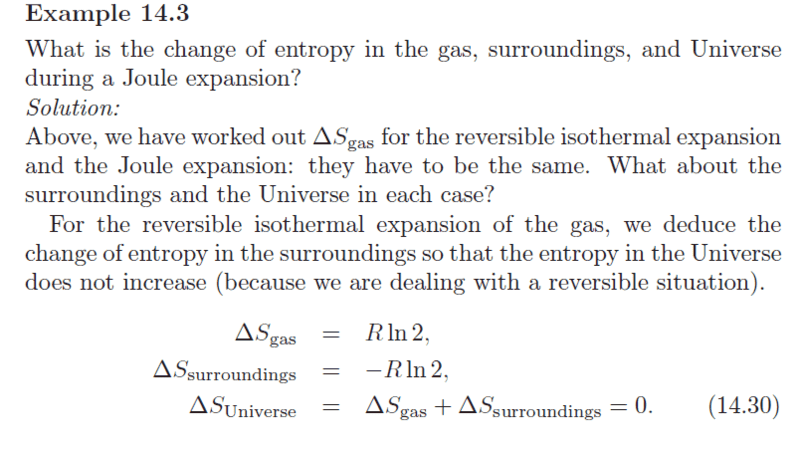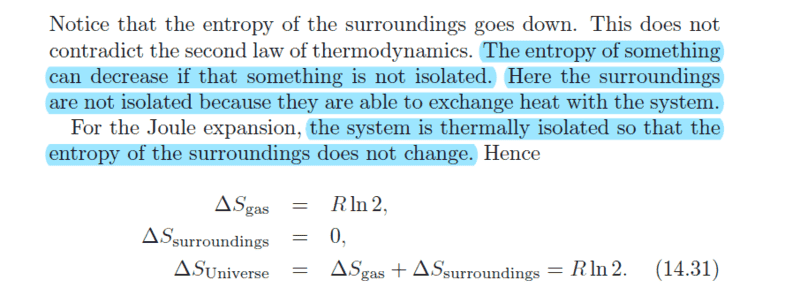After calculating the ## \Delta S## , we have to come back to our system, which is going through Joule Expansion; not the isothermal expansion.
Isothermal process is just a tool calculate ##\Delta S##.
Is this correct?

#### Attachments

Mentor
So, S is not a state- function
Look at the text after eq 14.2. S is a function of state.

Figure 14.1 explains the situation. Between any two states there are an infinite number of paths. For some paths you can calculate the change in entropy using the formula. For other paths you cannot calculate the change in entropy that way, but it is the same since it is an equation of state.

Mentor
I'm going to respond only to the last question of your original post, regarding the applicability of dU=TdS-PdV to an irreversible process. This equation describes the relationship between the changes in U, S, and V only between two closely neighboring thermodynamic equilibrium states of a system. So it can't be integrated along an arbitrary irreversible path and be expected to give the correct answer, as you have correctly noted. However, if a system starts out at a given thermodynamic equilibrium state and then, after suffering some wildly tortuous irreversible process path, ultimately finds itself situated in a closely neighboring thermodynamic equilibrium state to its original state, then the mutual differences in U, S, and V will indeed be correctly described by this equation.

Pushoam
Figure 14.1 explains the situation. Between any two states there are an infinite number of paths. For some paths you can calculate the change in entropy using the formula. For other paths you cannot calculate the change in entropy that way, but it is the same since it is an equation of state.
What I have understood is:
For calculating entropy - change, I have to consider only the final and initial state.
Then, I have to take a suitable reversible process which leads to the system to the final state from the initial state.
Then, I have to calculate entropy change using this reversible process.
It doesn't matter whether the system reaches to the final state through this reversible process, or some other reversible process, or some irreversible process.

Is this correct so far?

#### Attachments

Pushoam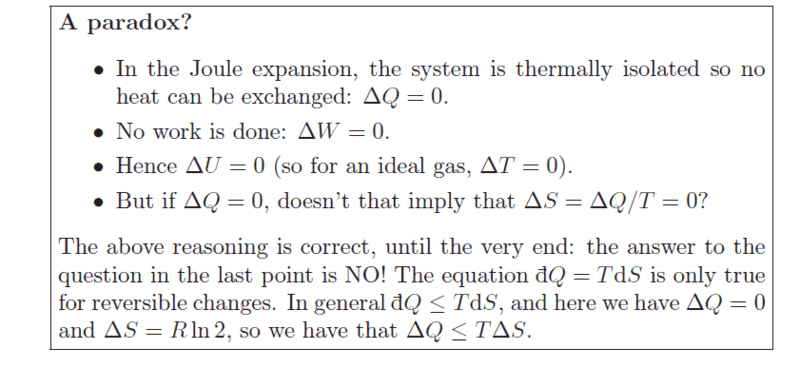Since the system is thermally isolated, the dQ is 0 for both reversible and irreversible process. So, entropy change should be 0.
What is wrong with the above argument?

Pushoam
However, if a system starts out at a given thermodynamic equilibrium state and then, after suffering some wildly tortuous irreversible process path, ultimately finds itself situated in a closely neighboring thermodynamic equilibrium state to its original state, then the mutual differences in U, S, and V will indeed be correctly described by this equation.
So what I understood is :
The eqn. holds true locally, not globally.
That is if the change in U,S,V are very small (i.e infinitesimally small) between the initial and final state, then the eqn. holds true.
Smaller the change in U,S,V, more correct it is to use the eqn.14.18.
Is this correct?
But how can one show that the eqn. hold true in this case?

Mentor
t doesn't matter whether the system reaches to the final state through this reversible process, or some other reversible process, or some irreversible process.
Yes

Mentor
So, entropy change should be 0.
Why? What equation leads to this conclusion?

Pushoam
Why? What equation leads to this conclusion?
Since the system is thermally isolated,dQ =0, irrespecctive which process( reversible or irreversible) it is going through.
##dS = \frac {dQ_{rev}} T = 0##

Mentor
Since the system is thermally isolated,dQ =0, irrespecctive which process( reversible or irreversible) it is going through
Yes, sort of. In Joule expansion the system is thermally isolated so dQ = 0. However, if you are considering a different path to a given state, then dQ=0 may not hold.

##dS = \frac {dQ_{rev}} T = 0##
Joule expansion is irreversible so this equation does not hold. Instead ##dS \ge \frac {dQ} T = 0##

Last edited:
Mentor
So what I understood is :
The eqn. holds true locally, not globally.
That is if the change in U,S,V are very small (i.e infinitesimally small) between the initial and final state, then the eqn. holds true.
Smaller the change in U,S,V, more correct it is to use the eqn.14.18.
Is this correct?
But how can one show that the eqn. hold true in this case?
The equation is correct for all reversible paths (which consist of a continuous sequence of thermodynamic equilibrium states), so it must be true in going differentially to a neighboring thermodynamics equilibrium state.

View attachment 212520
Since the system is thermally isolated, the dQ is 0 for both reversible and irreversible process. So, entropy change should be 0.

In an isolated system dQ = 0 because no heat is exchanged between the system and the environment. However, depending on the initial state immediately after isolation, even in isolated systems "internal things" can happen between the various parts of the system, e. g., reactions between components, redistribution of components by means of diffusion, phase transition etc., until the overall system has attained its absolute thermodynamic equilibrium state. That’s the general meaning of ΔSsystem >= 0 for isolated systems. Whatever happens inside an isolated system results in an increase of the system's entropy.

Since the system is thermally isolated,dQ =0, irrespecctive which process( reversible or irreversible) it is going through.
What you don't seem to realize is that the system is not thermally isolated in the reversible process which is used for the calculation (check it again).

The idea of the calculation is this: We don't know how to calculate things for the Joule expansion so let's pretend we have a different physical process with the same initial and final state as in the Joule expansion. So instead of the thermally isolated expansion (where no heat is exchanged), we calculate things for the reversible isothermal expansion (where heat is exchanged).

Last edited:
•Dale
Homework Helper
Gold Member
What I have understood is:
For calculating entropy - change, I have to consider only the final and initial state.
Then, I have to take a suitable reversible process which leads to the system to the final state from the initial state.
Then, I have to calculate entropy change using this reversible process.
It doesn't matter whether the system reaches to the final state through this reversible process, or some other reversible process, or some irreversible process.

Is this correct so far?
Yes.

•Chestermiller
Pushoam
Thank you.